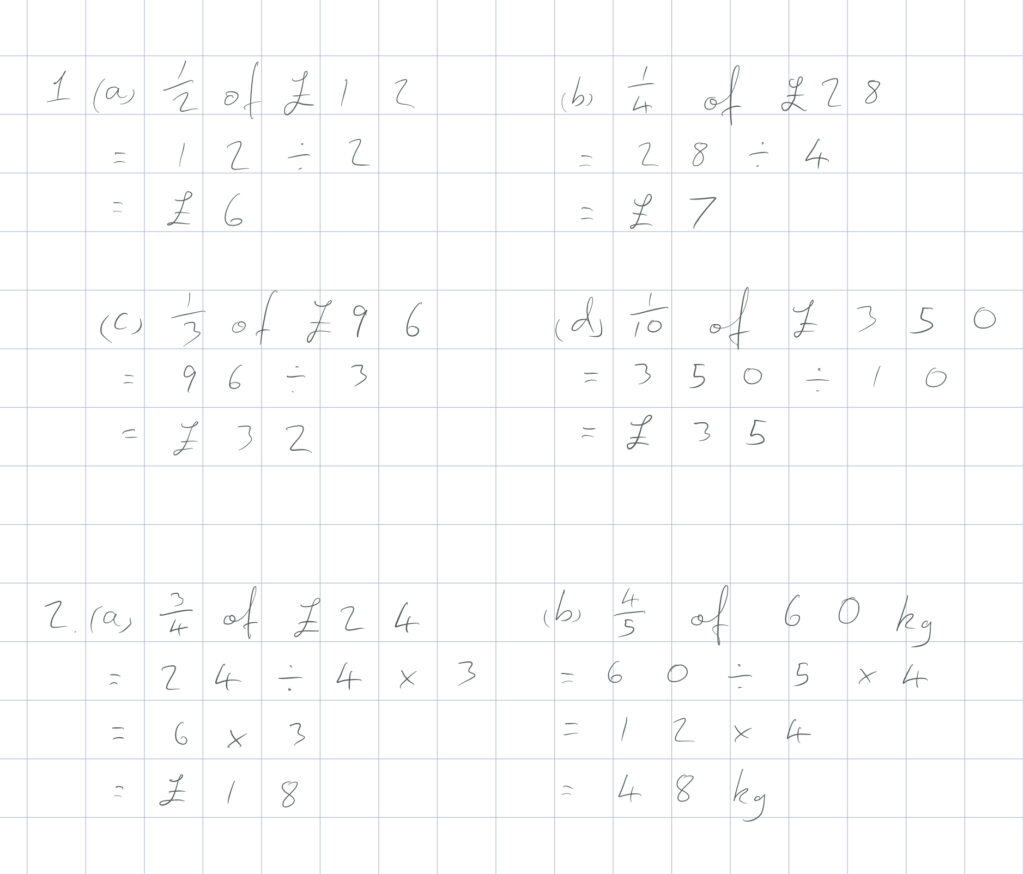There are many common fractions that we should be familiar with: 1/2, 1/4, 1/3, 1/5, 1/10, etc. To find an amount of a fraction where the numerator (top number) is 1, we divide by the denominator (as in question 1).

To find a fraction of any quantity, we can divide the quantity by the denominator (bottom number) and multiply it by the numerator (top number).